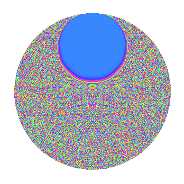# Properties

 Label 3.42.a.aLevel 3 Weight 42 Character orbit 3.a Self dual Yes Analytic conductor 31.942 Analytic rank 1 Dimension 3 CM No Inner twists 1

# Related objects

## Newspace parameters

 Level: $$N$$ = $$3$$ Weight: $$k$$ = $$42$$ Character orbit: $$[\chi]$$ = 3.a (trivial)

## Newform invariants

 Self dual: Yes Analytic conductor: $$31.9415011369$$ Analytic rank: $$1$$ Dimension: $$3$$ Coefficient field: $$\mathbb{Q}[x]/(x^{3} - \cdots)$$ Coefficient ring: $$\Z[a_1, \ldots, a_{5}]$$ Coefficient ring index: $$2^{10}\cdot 3^{5}\cdot 7$$ Fricke sign: $$1$$ Sato-Tate group: $\mathrm{SU}(2)$

## $q$-expansion

Coefficients of the $$q$$-expansion are expressed in terms of a basis $$1,\beta_1,\beta_2$$ for the coefficient ring described below. We also show the integral $$q$$-expansion of the trace form.

 $$f(q)$$ $$=$$ $$q$$ $$+ ( -96460 - \beta_{1} ) q^{2}$$ $$-3486784401 q^{3}$$ $$+ ( -751422059600 - 327756 \beta_{1} + 10 \beta_{2} ) q^{4}$$ $$+ ( 12883515397342 - 71244584 \beta_{1} + 2379 \beta_{2} ) q^{5}$$ $$+ ( 336335223320460 + 3486784401 \beta_{1} ) q^{6}$$ $$+ ( -14867403767136256 - 73320502584 \beta_{1} - 3812375 \beta_{2} ) q^{7}$$ $$+ ( 756010982896666432 + 2363361140656 \beta_{1} - 2893800 \beta_{2} ) q^{8}$$ $$+ 12157665459056928801 q^{9}$$ $$+O(q^{10})$$ $$q$$ $$+(-96460 - \beta_{1}) q^{2}$$ $$-3486784401 q^{3}$$ $$+(-751422059600 - 327756 \beta_{1} + 10 \beta_{2}) q^{4}$$ $$+(12883515397342 - 71244584 \beta_{1} + 2379 \beta_{2}) q^{5}$$ $$+(336335223320460 + 3486784401 \beta_{1}) q^{6}$$ $$+(-14867403767136256 - 73320502584 \beta_{1} - 3812375 \beta_{2}) q^{7}$$ $$+(756010982896666432 + 2363361140656 \beta_{1} - 2893800 \beta_{2}) q^{8}$$ $$+12157665459056928801 q^{9}$$ $$+($$$$10\!\cdots\!08$$$$- 149696474137566 \beta_{1} - 755720704 \beta_{2}) q^{10}$$ $$+($$$$72\!\cdots\!68$$$$+ 997689368410448 \beta_{1} + 8237865930 \beta_{2}) q^{11}$$ $$+($$$$26\!\cdots\!00$$$$+ 1142814508134156 \beta_{1} - 34867844010 \beta_{2}) q^{12}$$ $$+($$$$14\!\cdots\!14$$$$- 29481065101493616 \beta_{1} - 150407596430 \beta_{2}) q^{13}$$ $$+($$$$10\!\cdots\!28$$$$+ 154575166302522112 \beta_{1} + 3085958883840 \beta_{2}) q^{14}$$ $$+(-$$$$44\!\cdots\!42$$$$+ 248414504146934184 \beta_{1} - 8295060089979 \beta_{2}) q^{15}$$ $$+(-$$$$18\!\cdots\!32$$$$+ 1096962907304736576 \beta_{1} - 43837975805280 \beta_{2}) q^{16}$$ $$+(-$$$$36\!\cdots\!38$$$$+ 11928719000396751600 \beta_{1} + 216595399364670 \beta_{2}) q^{17}$$ $$+(-$$$$11\!\cdots\!60$$$$- 12157665459056928801 \beta_{1}) q^{18}$$ $$+($$$$65\!\cdots\!76$$$$+$$$$18\!\cdots\!28$$$$\beta_{1} - 634756701247710 \beta_{2}) q^{19}$$ $$+($$$$17\!\cdots\!08$$$$+ 25796271271357749784 \beta_{1} - 3268129131198804 \beta_{2}) q^{20}$$ $$+($$$$51\!\cdots\!56$$$$+$$$$25\!\cdots\!84$$$$\beta_{1} + 13292929680762375 \beta_{2}) q^{21}$$ $$+(-$$$$15\!\cdots\!76$$$$-$$$$66\!\cdots\!00$$$$\beta_{1} - 15060777312680960 \beta_{2}) q^{22}$$ $$+(-$$$$15\!\cdots\!28$$$$-$$$$17\!\cdots\!12$$$$\beta_{1} + 22177359062364210 \beta_{2}) q^{23}$$ $$+(-$$$$26\!\cdots\!32$$$$-$$$$82\!\cdots\!56$$$$\beta_{1} + 10090056699613800 \beta_{2}) q^{24}$$ $$+(-$$$$15\!\cdots\!33$$$$-$$$$64\!\cdots\!84$$$$\beta_{1} - 318019269759106796 \beta_{2}) q^{25}$$ $$+($$$$40\!\cdots\!92$$$$-$$$$20\!\cdots\!70$$$$\beta_{1} + 387632593445360640 \beta_{2}) q^{26}$$ $$-$$$$42\!\cdots\!01$$$$q^{27}$$ $$+(-$$$$19\!\cdots\!92$$$$-$$$$18\!\cdots\!68$$$$\beta_{1} + 4933293299122352640 \beta_{2}) q^{28}$$ $$+(-$$$$31\!\cdots\!38$$$$+$$$$65\!\cdots\!56$$$$\beta_{1} - 14886455450481587655 \beta_{2}) q^{29}$$ $$+(-$$$$35\!\cdots\!08$$$$+$$$$52\!\cdots\!66$$$$\beta_{1} + 2635035162219938304 \beta_{2}) q^{30}$$ $$+(-$$$$21\!\cdots\!36$$$$+$$$$83\!\cdots\!36$$$$\beta_{1} + 21546178470509132605 \beta_{2}) q^{31}$$ $$+(-$$$$30\!\cdots\!96$$$$-$$$$94\!\cdots\!64$$$$\beta_{1} + 22447897460436289920 \beta_{2}) q^{32}$$ $$+(-$$$$25\!\cdots\!68$$$$-$$$$34\!\cdots\!48$$$$\beta_{1} - 28723662422253357930 \beta_{2}) q^{33}$$ $$+(-$$$$16\!\cdots\!20$$$$-$$$$98\!\cdots\!62$$$$\beta_{1} -$$$$25\!\cdots\!20$$$$\beta_{2}) q^{34}$$ $$+(-$$$$51\!\cdots\!00$$$$-$$$$19\!\cdots\!00$$$$\beta_{1} +$$$$42\!\cdots\!50$$$$\beta_{2}) q^{35}$$ $$+(-$$$$91\!\cdots\!00$$$$-$$$$39\!\cdots\!56$$$$\beta_{1} +$$$$12\!\cdots\!10$$$$\beta_{2}) q^{36}$$ $$+(-$$$$14\!\cdots\!66$$$$+$$$$65\!\cdots\!56$$$$\beta_{1} -$$$$11\!\cdots\!20$$$$\beta_{2}) q^{37}$$ $$+(-$$$$27\!\cdots\!16$$$$+$$$$42\!\cdots\!72$$$$\beta_{1} -$$$$14\!\cdots\!20$$$$\beta_{2}) q^{38}$$ $$+(-$$$$51\!\cdots\!14$$$$+$$$$10\!\cdots\!16$$$$\beta_{1} +$$$$52\!\cdots\!30$$$$\beta_{2}) q^{39}$$ $$+(-$$$$27\!\cdots\!24$$$$+$$$$30\!\cdots\!48$$$$\beta_{1} +$$$$34\!\cdots\!12$$$$\beta_{2}) q^{40}$$ $$+(-$$$$14\!\cdots\!66$$$$+$$$$34\!\cdots\!96$$$$\beta_{1} +$$$$21\!\cdots\!70$$$$\beta_{2}) q^{41}$$ $$+(-$$$$37\!\cdots\!28$$$$-$$$$53\!\cdots\!12$$$$\beta_{1} -$$$$10\!\cdots\!40$$$$\beta_{2}) q^{42}$$ $$+(-$$$$43\!\cdots\!92$$$$-$$$$64\!\cdots\!64$$$$\beta_{1} -$$$$25\!\cdots\!90$$$$\beta_{2}) q^{43}$$ $$+(-$$$$48\!\cdots\!76$$$$-$$$$29\!\cdots\!20$$$$\beta_{1} -$$$$21\!\cdots\!00$$$$\beta_{2}) q^{44}$$ $$+($$$$15\!\cdots\!42$$$$-$$$$86\!\cdots\!84$$$$\beta_{1} +$$$$28\!\cdots\!79$$$$\beta_{2}) q^{45}$$ $$+($$$$26\!\cdots\!04$$$$-$$$$18\!\cdots\!64$$$$\beta_{1} +$$$$38\!\cdots\!60$$$$\beta_{2}) q^{46}$$ $$+($$$$21\!\cdots\!56$$$$-$$$$94\!\cdots\!48$$$$\beta_{1} -$$$$79\!\cdots\!90$$$$\beta_{2}) q^{47}$$ $$+($$$$63\!\cdots\!32$$$$-$$$$38\!\cdots\!76$$$$\beta_{1} +$$$$15\!\cdots\!80$$$$\beta_{2}) q^{48}$$ $$+($$$$57\!\cdots\!41$$$$+$$$$58\!\cdots\!52$$$$\beta_{1} -$$$$19\!\cdots\!40$$$$\beta_{2}) q^{49}$$ $$+($$$$94\!\cdots\!08$$$$+$$$$13\!\cdots\!09$$$$\beta_{1} +$$$$26\!\cdots\!96$$$$\beta_{2}) q^{50}$$ $$+($$$$12\!\cdots\!38$$$$-$$$$41\!\cdots\!00$$$$\beta_{1} -$$$$75\!\cdots\!70$$$$\beta_{2}) q^{51}$$ $$+(-$$$$69\!\cdots\!08$$$$-$$$$22\!\cdots\!80$$$$\beta_{1} +$$$$29\!\cdots\!20$$$$\beta_{2}) q^{52}$$ $$+($$$$11\!\cdots\!82$$$$-$$$$90\!\cdots\!32$$$$\beta_{1} +$$$$15\!\cdots\!85$$$$\beta_{2}) q^{53}$$ $$+($$$$40\!\cdots\!60$$$$+$$$$42\!\cdots\!01$$$$\beta_{1}) q^{54}$$ $$+($$$$33\!\cdots\!32$$$$+$$$$12\!\cdots\!36$$$$\beta_{1} +$$$$11\!\cdots\!84$$$$\beta_{2}) q^{55}$$ $$+(-$$$$18\!\cdots\!00$$$$-$$$$36\!\cdots\!20$$$$\beta_{1} -$$$$96\!\cdots\!40$$$$\beta_{2}) q^{56}$$ $$+(-$$$$22\!\cdots\!76$$$$-$$$$65\!\cdots\!28$$$$\beta_{1} +$$$$22\!\cdots\!10$$$$\beta_{2}) q^{57}$$ $$+(-$$$$91\!\cdots\!32$$$$+$$$$12\!\cdots\!34$$$$\beta_{1} +$$$$26\!\cdots\!20$$$$\beta_{2}) q^{58}$$ $$+(-$$$$15\!\cdots\!16$$$$-$$$$49\!\cdots\!48$$$$\beta_{1} +$$$$18\!\cdots\!40$$$$\beta_{2}) q^{59}$$ $$+(-$$$$61\!\cdots\!08$$$$-$$$$89\!\cdots\!84$$$$\beta_{1} +$$$$11\!\cdots\!04$$$$\beta_{2}) q^{60}$$ $$+(-$$$$28\!\cdots\!54$$$$+$$$$15\!\cdots\!52$$$$\beta_{1} -$$$$40\!\cdots\!40$$$$\beta_{2}) q^{61}$$ $$+($$$$88\!\cdots\!88$$$$+$$$$12\!\cdots\!12$$$$\beta_{1} -$$$$14\!\cdots\!40$$$$\beta_{2}) q^{62}$$ $$+(-$$$$18\!\cdots\!56$$$$-$$$$89\!\cdots\!84$$$$\beta_{1} -$$$$46\!\cdots\!75$$$$\beta_{2}) q^{63}$$ $$+($$$$56\!\cdots\!52$$$$-$$$$75\!\cdots\!80$$$$\beta_{1} +$$$$92\!\cdots\!80$$$$\beta_{2}) q^{64}$$ $$+($$$$90\!\cdots\!16$$$$-$$$$60\!\cdots\!32$$$$\beta_{1} +$$$$39\!\cdots\!42$$$$\beta_{2}) q^{65}$$ $$+($$$$52\!\cdots\!76$$$$+$$$$23\!\cdots\!00$$$$\beta_{1} +$$$$52\!\cdots\!60$$$$\beta_{2}) q^{66}$$ $$+($$$$10\!\cdots\!72$$$$-$$$$16\!\cdots\!60$$$$\beta_{1} +$$$$14\!\cdots\!00$$$$\beta_{2}) q^{67}$$ $$+($$$$11\!\cdots\!00$$$$+$$$$14\!\cdots\!28$$$$\beta_{1} -$$$$31\!\cdots\!00$$$$\beta_{2}) q^{68}$$ $$+($$$$54\!\cdots\!28$$$$+$$$$60\!\cdots\!12$$$$\beta_{1} -$$$$77\!\cdots\!10$$$$\beta_{2}) q^{69}$$ $$+($$$$32\!\cdots\!00$$$$+$$$$23\!\cdots\!00$$$$\beta_{1} -$$$$68\!\cdots\!00$$$$\beta_{2}) q^{70}$$ $$+($$$$49\!\cdots\!32$$$$+$$$$27\!\cdots\!40$$$$\beta_{1} +$$$$90\!\cdots\!10$$$$\beta_{2}) q^{71}$$ $$+($$$$91\!\cdots\!32$$$$+$$$$28\!\cdots\!56$$$$\beta_{1} -$$$$35\!\cdots\!00$$$$\beta_{2}) q^{72}$$ $$+($$$$22\!\cdots\!82$$$$-$$$$12\!\cdots\!92$$$$\beta_{1} -$$$$10\!\cdots\!00$$$$\beta_{2}) q^{73}$$ $$+($$$$48\!\cdots\!48$$$$+$$$$15\!\cdots\!62$$$$\beta_{1} +$$$$79\!\cdots\!60$$$$\beta_{2}) q^{74}$$ $$+($$$$53\!\cdots\!33$$$$+$$$$22\!\cdots\!84$$$$\beta_{1} +$$$$11\!\cdots\!96$$$$\beta_{2}) q^{75}$$ $$+(-$$$$17\!\cdots\!36$$$$-$$$$51\!\cdots\!88$$$$\beta_{1} +$$$$18\!\cdots\!20$$$$\beta_{2}) q^{76}$$ $$+(-$$$$31\!\cdots\!72$$$$-$$$$30\!\cdots\!68$$$$\beta_{1} -$$$$44\!\cdots\!20$$$$\beta_{2}) q^{77}$$ $$+(-$$$$14\!\cdots\!92$$$$+$$$$72\!\cdots\!70$$$$\beta_{1} -$$$$13\!\cdots\!40$$$$\beta_{2}) q^{78}$$ $$+(-$$$$37\!\cdots\!00$$$$-$$$$27\!\cdots\!80$$$$\beta_{1} +$$$$32\!\cdots\!65$$$$\beta_{2}) q^{79}$$ $$+(-$$$$80\!\cdots\!92$$$$+$$$$19\!\cdots\!84$$$$\beta_{1} +$$$$19\!\cdots\!96$$$$\beta_{2}) q^{80}$$ $$+$$$$14\!\cdots\!01$$$$q^{81}$$ $$+(-$$$$35\!\cdots\!32$$$$+$$$$66\!\cdots\!02$$$$\beta_{1} -$$$$16\!\cdots\!80$$$$\beta_{2}) q^{82}$$ $$+(-$$$$39\!\cdots\!20$$$$+$$$$26\!\cdots\!52$$$$\beta_{1} +$$$$15\!\cdots\!50$$$$\beta_{2}) q^{83}$$ $$+($$$$69\!\cdots\!92$$$$+$$$$63\!\cdots\!68$$$$\beta_{1} -$$$$17\!\cdots\!40$$$$\beta_{2}) q^{84}$$ $$+($$$$20\!\cdots\!84$$$$+$$$$26\!\cdots\!32$$$$\beta_{1} -$$$$30\!\cdots\!42$$$$\beta_{2}) q^{85}$$ $$+($$$$96\!\cdots\!48$$$$+$$$$17\!\cdots\!68$$$$\beta_{1} +$$$$65\!\cdots\!80$$$$\beta_{2}) q^{86}$$ $$+($$$$10\!\cdots\!38$$$$-$$$$22\!\cdots\!56$$$$\beta_{1} +$$$$51\!\cdots\!55$$$$\beta_{2}) q^{87}$$ $$+($$$$37\!\cdots\!52$$$$+$$$$19\!\cdots\!56$$$$\beta_{1} +$$$$37\!\cdots\!20$$$$\beta_{2}) q^{88}$$ $$+(-$$$$96\!\cdots\!34$$$$-$$$$78\!\cdots\!32$$$$\beta_{1} -$$$$68\!\cdots\!80$$$$\beta_{2}) q^{89}$$ $$+($$$$12\!\cdots\!08$$$$-$$$$18\!\cdots\!66$$$$\beta_{1} -$$$$91\!\cdots\!04$$$$\beta_{2}) q^{90}$$ $$+($$$$65\!\cdots\!04$$$$+$$$$50\!\cdots\!76$$$$\beta_{1} +$$$$55\!\cdots\!90$$$$\beta_{2}) q^{91}$$ $$+($$$$34\!\cdots\!44$$$$+$$$$93\!\cdots\!96$$$$\beta_{1} -$$$$49\!\cdots\!40$$$$\beta_{2}) q^{92}$$ $$+($$$$75\!\cdots\!36$$$$-$$$$29\!\cdots\!36$$$$\beta_{1} -$$$$75\!\cdots\!05$$$$\beta_{2}) q^{93}$$ $$+($$$$11\!\cdots\!36$$$$-$$$$21\!\cdots\!24$$$$\beta_{1} +$$$$14\!\cdots\!20$$$$\beta_{2}) q^{94}$$ $$+(-$$$$28\!\cdots\!32$$$$+$$$$23\!\cdots\!64$$$$\beta_{1} +$$$$33\!\cdots\!16$$$$\beta_{2}) q^{95}$$ $$+($$$$10\!\cdots\!96$$$$+$$$$33\!\cdots\!64$$$$\beta_{1} -$$$$78\!\cdots\!20$$$$\beta_{2}) q^{96}$$ $$+(-$$$$17\!\cdots\!38$$$$+$$$$39\!\cdots\!00$$$$\beta_{1} -$$$$40\!\cdots\!80$$$$\beta_{2}) q^{97}$$ $$+(-$$$$89\!\cdots\!64$$$$-$$$$23\!\cdots\!09$$$$\beta_{1} -$$$$46\!\cdots\!80$$$$\beta_{2}) q^{98}$$ $$+($$$$87\!\cdots\!68$$$$+$$$$12\!\cdots\!48$$$$\beta_{1} +$$$$10\!\cdots\!30$$$$\beta_{2}) q^{99}$$ $$+O(q^{100})$$ $$\operatorname{Tr}(f)(q)$$ $$=$$ $$3q$$ $$\mathstrut -\mathstrut 289380q^{2}$$ $$\mathstrut -\mathstrut 10460353203q^{3}$$ $$\mathstrut -\mathstrut 2254266178800q^{4}$$ $$\mathstrut +\mathstrut 38650546192026q^{5}$$ $$\mathstrut +\mathstrut 1009005669961380q^{6}$$ $$\mathstrut -\mathstrut 44602211301408768q^{7}$$ $$\mathstrut +\mathstrut 2268032948689999296q^{8}$$ $$\mathstrut +\mathstrut 36472996377170786403q^{9}$$ $$\mathstrut +\mathstrut O(q^{10})$$ $$3q$$ $$\mathstrut -\mathstrut 289380q^{2}$$ $$\mathstrut -\mathstrut 10460353203q^{3}$$ $$\mathstrut -\mathstrut 2254266178800q^{4}$$ $$\mathstrut +\mathstrut 38650546192026q^{5}$$ $$\mathstrut +\mathstrut 1009005669961380q^{6}$$ $$\mathstrut -\mathstrut 44602211301408768q^{7}$$ $$\mathstrut +\mathstrut 2268032948689999296q^{8}$$ $$\mathstrut +\mathstrut 36472996377170786403q^{9}$$ $$\mathstrut +\mathstrut$$$$30\!\cdots\!24$$$$q^{10}$$ $$\mathstrut +\mathstrut$$$$21\!\cdots\!04$$$$q^{11}$$ $$\mathstrut +\mathstrut$$$$78\!\cdots\!00$$$$q^{12}$$ $$\mathstrut +\mathstrut$$$$44\!\cdots\!42$$$$q^{13}$$ $$\mathstrut +\mathstrut$$$$32\!\cdots\!84$$$$q^{14}$$ $$\mathstrut -\mathstrut$$$$13\!\cdots\!26$$$$q^{15}$$ $$\mathstrut -\mathstrut$$$$54\!\cdots\!96$$$$q^{16}$$ $$\mathstrut -\mathstrut$$$$10\!\cdots\!14$$$$q^{17}$$ $$\mathstrut -\mathstrut$$$$35\!\cdots\!80$$$$q^{18}$$ $$\mathstrut +\mathstrut$$$$19\!\cdots\!28$$$$q^{19}$$ $$\mathstrut +\mathstrut$$$$53\!\cdots\!24$$$$q^{20}$$ $$\mathstrut +\mathstrut$$$$15\!\cdots\!68$$$$q^{21}$$ $$\mathstrut -\mathstrut$$$$45\!\cdots\!28$$$$q^{22}$$ $$\mathstrut -\mathstrut$$$$46\!\cdots\!84$$$$q^{23}$$ $$\mathstrut -\mathstrut$$$$79\!\cdots\!96$$$$q^{24}$$ $$\mathstrut -\mathstrut$$$$46\!\cdots\!99$$$$q^{25}$$ $$\mathstrut +\mathstrut$$$$12\!\cdots\!76$$$$q^{26}$$ $$\mathstrut -\mathstrut$$$$12\!\cdots\!03$$$$q^{27}$$ $$\mathstrut -\mathstrut$$$$59\!\cdots\!76$$$$q^{28}$$ $$\mathstrut -\mathstrut$$$$94\!\cdots\!14$$$$q^{29}$$ $$\mathstrut -\mathstrut$$$$10\!\cdots\!24$$$$q^{30}$$ $$\mathstrut -\mathstrut$$$$64\!\cdots\!08$$$$q^{31}$$ $$\mathstrut -\mathstrut$$$$91\!\cdots\!88$$$$q^{32}$$ $$\mathstrut -\mathstrut$$$$75\!\cdots\!04$$$$q^{33}$$ $$\mathstrut -\mathstrut$$$$50\!\cdots\!60$$$$q^{34}$$ $$\mathstrut -\mathstrut$$$$15\!\cdots\!00$$$$q^{35}$$ $$\mathstrut -\mathstrut$$$$27\!\cdots\!00$$$$q^{36}$$ $$\mathstrut -\mathstrut$$$$44\!\cdots\!98$$$$q^{37}$$ $$\mathstrut -\mathstrut$$$$83\!\cdots\!48$$$$q^{38}$$ $$\mathstrut -\mathstrut$$$$15\!\cdots\!42$$$$q^{39}$$ $$\mathstrut -\mathstrut$$$$83\!\cdots\!72$$$$q^{40}$$ $$\mathstrut -\mathstrut$$$$43\!\cdots\!98$$$$q^{41}$$ $$\mathstrut -\mathstrut$$$$11\!\cdots\!84$$$$q^{42}$$ $$\mathstrut -\mathstrut$$$$13\!\cdots\!76$$$$q^{43}$$ $$\mathstrut -\mathstrut$$$$14\!\cdots\!28$$$$q^{44}$$ $$\mathstrut +\mathstrut$$$$46\!\cdots\!26$$$$q^{45}$$ $$\mathstrut +\mathstrut$$$$79\!\cdots\!12$$$$q^{46}$$ $$\mathstrut +\mathstrut$$$$64\!\cdots\!68$$$$q^{47}$$ $$\mathstrut +\mathstrut$$$$19\!\cdots\!96$$$$q^{48}$$ $$\mathstrut +\mathstrut$$$$17\!\cdots\!23$$$$q^{49}$$ $$\mathstrut +\mathstrut$$$$28\!\cdots\!24$$$$q^{50}$$ $$\mathstrut +\mathstrut$$$$38\!\cdots\!14$$$$q^{51}$$ $$\mathstrut -\mathstrut$$$$20\!\cdots\!24$$$$q^{52}$$ $$\mathstrut +\mathstrut$$$$34\!\cdots\!46$$$$q^{53}$$ $$\mathstrut +\mathstrut$$$$12\!\cdots\!80$$$$q^{54}$$ $$\mathstrut +\mathstrut$$$$10\!\cdots\!96$$$$q^{55}$$ $$\mathstrut -\mathstrut$$$$56\!\cdots\!00$$$$q^{56}$$ $$\mathstrut -\mathstrut$$$$68\!\cdots\!28$$$$q^{57}$$ $$\mathstrut -\mathstrut$$$$27\!\cdots\!96$$$$q^{58}$$ $$\mathstrut -\mathstrut$$$$45\!\cdots\!48$$$$q^{59}$$ $$\mathstrut -\mathstrut$$$$18\!\cdots\!24$$$$q^{60}$$ $$\mathstrut -\mathstrut$$$$86\!\cdots\!62$$$$q^{61}$$ $$\mathstrut +\mathstrut$$$$26\!\cdots\!64$$$$q^{62}$$ $$\mathstrut -\mathstrut$$$$54\!\cdots\!68$$$$q^{63}$$ $$\mathstrut +\mathstrut$$$$16\!\cdots\!56$$$$q^{64}$$ $$\mathstrut +\mathstrut$$$$27\!\cdots\!48$$$$q^{65}$$ $$\mathstrut +\mathstrut$$$$15\!\cdots\!28$$$$q^{66}$$ $$\mathstrut +\mathstrut$$$$32\!\cdots\!16$$$$q^{67}$$ $$\mathstrut +\mathstrut$$$$33\!\cdots\!00$$$$q^{68}$$ $$\mathstrut +\mathstrut$$$$16\!\cdots\!84$$$$q^{69}$$ $$\mathstrut +\mathstrut$$$$98\!\cdots\!00$$$$q^{70}$$ $$\mathstrut +\mathstrut$$$$14\!\cdots\!96$$$$q^{71}$$ $$\mathstrut +\mathstrut$$$$27\!\cdots\!96$$$$q^{72}$$ $$\mathstrut +\mathstrut$$$$66\!\cdots\!46$$$$q^{73}$$ $$\mathstrut +\mathstrut$$$$14\!\cdots\!44$$$$q^{74}$$ $$\mathstrut +\mathstrut$$$$16\!\cdots\!99$$$$q^{75}$$ $$\mathstrut -\mathstrut$$$$53\!\cdots\!08$$$$q^{76}$$ $$\mathstrut -\mathstrut$$$$95\!\cdots\!16$$$$q^{77}$$ $$\mathstrut -\mathstrut$$$$42\!\cdots\!76$$$$q^{78}$$ $$\mathstrut -\mathstrut$$$$11\!\cdots\!00$$$$q^{79}$$ $$\mathstrut -\mathstrut$$$$24\!\cdots\!76$$$$q^{80}$$ $$\mathstrut +\mathstrut$$$$44\!\cdots\!03$$$$q^{81}$$ $$\mathstrut -\mathstrut$$$$10\!\cdots\!96$$$$q^{82}$$ $$\mathstrut -\mathstrut$$$$11\!\cdots\!60$$$$q^{83}$$ $$\mathstrut +\mathstrut$$$$20\!\cdots\!76$$$$q^{84}$$ $$\mathstrut +\mathstrut$$$$61\!\cdots\!52$$$$q^{85}$$ $$\mathstrut +\mathstrut$$$$28\!\cdots\!44$$$$q^{86}$$ $$\mathstrut +\mathstrut$$$$32\!\cdots\!14$$$$q^{87}$$ $$\mathstrut +\mathstrut$$$$11\!\cdots\!56$$$$q^{88}$$ $$\mathstrut -\mathstrut$$$$29\!\cdots\!02$$$$q^{89}$$ $$\mathstrut +\mathstrut$$$$36\!\cdots\!24$$$$q^{90}$$ $$\mathstrut +\mathstrut$$$$19\!\cdots\!12$$$$q^{91}$$ $$\mathstrut +\mathstrut$$$$10\!\cdots\!32$$$$q^{92}$$ $$\mathstrut +\mathstrut$$$$22\!\cdots\!08$$$$q^{93}$$ $$\mathstrut +\mathstrut$$$$34\!\cdots\!08$$$$q^{94}$$ $$\mathstrut -\mathstrut$$$$84\!\cdots\!96$$$$q^{95}$$ $$\mathstrut +\mathstrut$$$$32\!\cdots\!88$$$$q^{96}$$ $$\mathstrut -\mathstrut$$$$52\!\cdots\!14$$$$q^{97}$$ $$\mathstrut -\mathstrut$$$$26\!\cdots\!92$$$$q^{98}$$ $$\mathstrut +\mathstrut$$$$26\!\cdots\!04$$$$q^{99}$$ $$\mathstrut +\mathstrut O(q^{100})$$

Basis of coefficient ring in terms of a root $$\nu$$ of $$x^{3}\mathstrut -\mathstrut$$ $$x^{2}\mathstrut -\mathstrut$$ $$14982256920$$ $$x\mathstrut +\mathstrut$$ $$433388802120300$$:

 $$\beta_{0}$$ $$=$$ $$1$$ $$\beta_{1}$$ $$=$$ $$12 \nu - 4$$ $$\beta_{2}$$ $$=$$ $$($$$$72 \nu^{2} + 3124008 \nu - 719149373520$$$$)/5$$
 $$1$$ $$=$$ $$\beta_0$$ $$\nu$$ $$=$$ $$($$$$\beta_{1}\mathstrut +\mathstrut$$ $$4$$$$)/12$$ $$\nu^{2}$$ $$=$$ $$($$$$5$$ $$\beta_{2}\mathstrut -\mathstrut$$ $$260334$$ $$\beta_{1}\mathstrut +\mathstrut$$ $$719148332184$$$$)/72$$

## Embeddings

For each embedding $$\iota_m$$ of the coefficient field, the values $$\iota_m(a_n)$$ are shown below.

For more information on an embedded modular form you can click on its label.

Label $$\iota_m(\nu)$$ $$a_{2}$$ $$a_{3}$$ $$a_{4}$$ $$a_{5}$$ $$a_{6}$$ $$a_{7}$$ $$a_{8}$$ $$a_{9}$$ $$a_{10}$$
1.1
 103995. 30895.0 −134889.
−1.34440e6 −3.48678e9 −3.91623e11 1.06877e14 4.68762e15 −3.99469e17 3.48285e18 1.21577e19 −1.43685e20
1.2 −467196. −3.48678e9 −1.98075e12 −2.77079e14 1.62901e15 3.80292e17 1.95278e18 1.21577e19 1.29450e20
1.3 1.52221e6 −3.48678e9 1.18107e11 2.08853e14 −5.30763e15 −2.54249e16 −3.16760e18 1.21577e19 3.17919e20
 $$n$$: e.g. 2-40 or 990-1000 Significant digits: Format: Complex embeddings Normalized embeddings Satake parameters Satake angles

## Inner twists

This newform does not admit any (nontrivial) inner twists.

## Atkin-Lehner signs

$$p$$ Sign
$$3$$ $$1$$

## Hecke kernels

This newform can be constructed as the kernel of the linear operator $$T_{2}^{3}$$ $$\mathstrut +\mathstrut 289380 T_{2}^{2}$$ $$\mathstrut -\mathstrut$$$$21\!\cdots\!28$$$$T_{2}$$ $$\mathstrut -\mathstrut$$$$95\!\cdots\!32$$ acting on $$S_{42}^{\mathrm{new}}(\Gamma_0(3))$$.